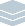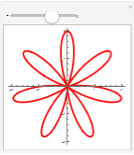Wolfram Archive
Wolfram Programming Lab is a legacy product.
All the same functionality and features, including access to Programming Lab Explorations, are available with Wolfram|One.
Start programming now. »
Try it now »
(no sign-in required)# Heart and Flower Curves

Use polar plots to make natural-looking shapes.

Run the code to make a heart-like (cardioid) plot. Try values other than 0.5for example, 1 or 4:

PolarPlot plots curves in polar coordinates.

This plots the path of a point that has a constant distance of 1 unit from the origin as an angle sweeps from 0 to 360 degrees:

PolarPlot[1, {angle, 0 Degree, 360 Degree}]

Drag the slider to see how the curve is plotted:

This plots the path of a point whose distance from the origin is proportional to the angle:

PolarPlot[angle, {angle, 0 Degree, 360 Degree}]

Drag the slider to see how this curve is plotted:

This plots a heart shape (cardioid):

PolarPlot[1 - Sin[0.5 angle], {angle, 0 Degree, 360 Degree}]

Drag the slider to see how the cardioid is plotted:

PolarPlot[1 - Sin[0.5 angle], {angle, 0 Degree, 360 Degree}]

Allow the parameter to change. Drag the slider to get different shapes:

Hint: press (Windows) or (Mac) while dragging the slider for fine adjustment.

Note: click the + to the right of the slider to see the value of the parameter.

Add a slider to control the value of the parameter with Manipulate.

Replace the parameter 0.5 with the variable n, wrap the PolarPlot expression with Manipulate, and specify that n varies from 0 to 10, with an initial value of 0.5. Now you can drag the slider to change the value of the parameter:

Manipulate[ PolarPlot[1 - Sin[n angle], {angle, 0 Degree, 360 Degree}], {{n, 0.5}, 0, 10} ]

Add a plot range to keep the plot from jumping around as you drag the slider:

Manipulate[ PolarPlot[1 - Sin[n angle], {angle, 0 Degree, 360 Degree}, PlotRange -> 2], {{n, 0.5}, 0, 10} ]

Manipulate[ PolarPlot[1 - Sin[n angle], {angle, 0 Degree, 360 Degree}, PlotRange -> 2], {{n, 0.5}, 0, 10} ]

Make the curve thick and red. Try other colors, like Blue or Green:

Hint: color names are capitalizedfor example, Purple, Gray, and Green.

PolarPlot normally draws a blue curve:

PolarPlot[1, {angle, 0 Degree, 360 Degree}]

You can change the color of the curve with PlotStyle:

PolarPlot[1, {angle, 0 Degree, 360 Degree}, PlotStyle -> Red]

Make the curve thicker with AbsoluteThickness. If there is more than one style element, put the elements in a list ({}):

PolarPlot[1, {angle, 0 Degree, 360 Degree}, PlotStyle -> {Red, AbsoluteThickness}]

Manipulate[ PolarPlot[1 - Sin[n angle], {angle, 0 Degree, 360 Degree}, PlotRange -> 2, PlotStyle -> {Red, AbsoluteThickness}], {{n, 0.5}, 0, 10}]

Share ItMake an interactive website for exploring heart and flower curves:

Deploy the Manipulate to the Wolfram Cloud where anyone with a browser can use it:

CloudDeploy[ Manipulate[ PolarPlot[1 - Sin[n angle], {angle, 0 Degree, 360 Degree}, PlotRange -> 2, PlotStyle -> {Red, AbsoluteThickness}], {{n, 0.5}, 0, 10}], Permissions -> "Public" ]

Click on the link in the output to visit the site.